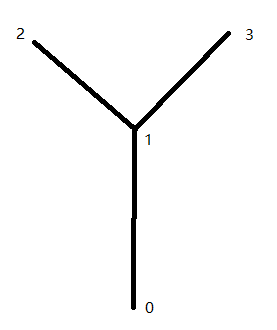# VMTK: Problem about multiple source/target points for vtkvmtkPolyDataCenterlines

I am using vmtk to extract center lines from airway, and I need some help. For example, the airway looks like:If the source point is 0, and target point is 1, the output is OK:

If the source point is [1, 1], and target point is [2, 3], the output is also acceptable:

However, if I want to extract the three center lines, and set the source point as [0, 1, 1], target point is [1, 2, 3], the output is:

The line [0, 1] is missed. What is the reason to cause this problem?

Moreover, if I separately extract line (0, 1) and (1,2)/(1,3), the computation time would be double. How can I reduce the computation time for this situation?

Any suggestion is appreciated!

The question got answered here: https://github.com/vmtk/vmtk/issues/355